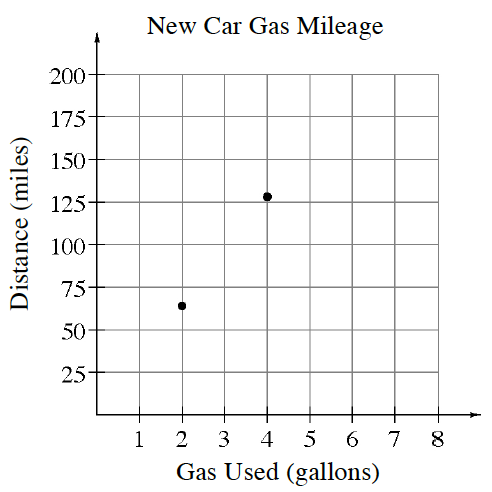### Home > ACC6 > Chapter 7 Unit 7 > Lesson CC1: 7.2.3 > Problem7-68

7-68.

The graph below displays gas mileage for a new car.

1. Use the graph to predict the number of miles the car could travel with three gallons of gas.

A line drawn through the two points would cross $3$ at about $100$ miles, so that is a reasonable estimate.

2. Use the graph to predict the number of miles the car could travel with five gallons of gas.

Where does the line from part (a) cross $5$ gallons?Use the eTool to continue graphing points by adding point to the table.
Click on the link at right for the full eTool version: